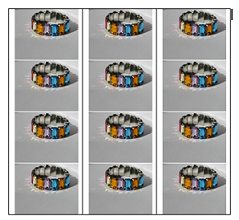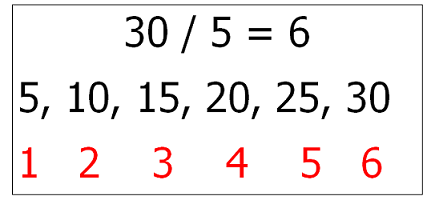# Division Lesson for Kids: Definition & Method

Instructor: Audrey Akins

Audrey has more than a decade of experience teaching elementary. She has a bachelor's in journalism and a master's in education.

Traditionally people believed that they had to know how to multiply before they could divide, but there are more strategies. Strategies such as drawing pictures or using objects, skip counting, and subtracting are all ways we'll look at using in this lesson about division.

## Parts of a Division Problem

Before you can understand how to divide, you must first understand the parts of a division problem. The dividend is the big number you're dividing. The divisor is the number of parts you're dividing the dividend into. The quotient is the answer you get when you divide.

## Draw a Picture or Use Objects

When someone has to divide, they usually are separating a group of things into equal shares. In fact, some people think of dividing as sharing equally. One strategy that is commonly used when first learning to divide is using a picture or counting objects into equal groups. If you had 12 bracelets to put on 3 dolls, you could divide by counting the bracelets out to each doll one by one until all the bracelets are gone. By doing this, you would see that each doll would get 4 bracelets so 12 / 3 = 4. In this case, 12 is the dividend, 3 is the divisor, and 4 is the quotient.## Skip Count

By now you probably know how to skip count, so when it comes to dividing you can use that skill to help you. Here's how: If you went on a field trip to a pumpkin patch and collected 30 pumpkins and wanted to divide them between 5 of your friends, you could skip count by 5 to determine how many pumpkins each friend would get. You would skip count by the number divisor and stop when you get to the dividend, so you would say 5, 10, 15, 20, 25, 30. You said six numbers when you were skip counting so 30 / 5 = 6.## Subtracting

Another skill that you already have that you can rely on to divide is subtraction. You can keep subtracting the divisor from the dividend until you get to zero. Then count the number of times you subtracted. This will be your quotient. For example, if you brought 42 cupcakes to school to share with 7 classes at your school, you would need to divide in order to know how many cupcakes each class gets. You could subtract 7 from 42 until you get to 0.

Let's try it.

42 - 7 = 35

35 - 7 = 28

28 - 7 = 21

21 - 7 = 14

14 -7 = 7

7 - 7 = 0

To unlock this lesson you must be a Study.com Member.

### Register to view this lesson

Are you a student or a teacher?

#### See for yourself why 30 million people use Study.com

##### Become a Study.com member and start learning now.
Back
What teachers are saying about Study.com

### Earning College Credit

Did you know… We have over 200 college courses that prepare you to earn credit by exam that is accepted by over 1,500 colleges and universities. You can test out of the first two years of college and save thousands off your degree. Anyone can earn credit-by-exam regardless of age or education level.